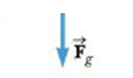Chapter 8, Problem 33P

Chapter
Section
Textbook Problem

The chewing muscle, the masseter, is one of the strongest in the human body. It is attached to the mandible (lower jawbone) as shown in Figure P8.33a. The jawbone is pivoted about a socket just in front of the auditory canal. The forces acting on the jawbone are equivalent to those acting on the curved bar in Figure P8.33b. F → C is the force exerted by the food being chewed against the jawbone, T → is the force of tension in the masseter, and R → is the force exerted by the socket on the mandible. Find T → and R → for a person who bites down on a piece of steak with a force of 50.0 N.Figure P8.32Figure P8.33

To determine
The force of tension and the forced exerted by the socket on the mandible.

Explanation

Given info: The force exerted by the food being chewed against jaw is 50.0N , the distance between the forces FC and T is 7.50cm , distance between the force T and the force R is 3.50cm and the force R is 107N .

Explanation: The torque about an axis that perpendicular to the page through the point where the tension force acts is τ=0=l1FCl2R . The sum of normal forces on the system is Fy=0=Fcl2R and from which the force of tension is calculated.

The formula for the force R is,

R=l1FCl2

• l1 is distance between the forces FC and T .
• l2 is distance between the force T and the force R .
• FC is force exerted by the food being chewed against jaw

Still sussing out bartleby?

Check out a sample textbook solution.

See a sample solution

The Solution to Your Study Problems

Bartleby provides explanations to thousands of textbook problems written by our experts, many with advanced degrees!

Get Started

Find more solutions based on key concepts